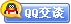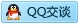##用户名 Email 自动登录 找回密码 密码 会员注册
 VIP会员，3年作业免费下 ！ 奥鹏作业，奥鹏毕业论文检测 新手作业下载教程，充值问题 没有找到答案，请在此处留言！ 2019年12月最新全国统考资料 投诉建议，加盟合作！奥鹏课程积分软件(ver:3.1)

# 东大19秋学期《电路原理》在线平时作业1（参考）发表于 2019-9-7 03:01:05 | 显示全部楼层 |阅读模式【奥鹏】[东北大学]19秋学期《电路原理》在线平时作业1 试卷总分:100    得分:100 第1题,图中t30时uc(t)为(      )。A u(t)=12e-0.25t     B u(t)=12-12e-0.25t    C 其它 A、A B、B C、C D、D 正确答案: 第2题,图中t30时iL(t) 为(      )。A iL(t)=3-3e-4t     B iL(t)=3+3e-4t    C 其它 A、A B、B C、C D、D 正确答案: 第3题,图中所示为矩形脉冲，试求其象函数F（S）为（      ）。 A (1/S)(1-e-St0)                  B 1/S                    C其它 A、A B、B C、C D、D 正确答案: 第4题,如图理想变压器的电流方程为(      )。A i1=ni2 ,         B i1=-(1/n)i2 ,       C 其它 A、A B、B C、C D、D 正确答案: 第5题,图中所示电路C=0.1F,R=10Ω,求冲击响应u为（      ） 。 A 0               B e-t1(t) V                  C其它 A、A B、B C、C D、D 正确答案: 第6题,电流表A1的读数为5A， A2为20A， A3为25A，电流表A4的读数为45A 。（      ） A、错误 B、正确 正确答案: 第7题,图示电路，t=0 时将开关K闭合，t0时电路已达稳态，电流i2(0)=5 mA 。(      ) A、错误 B、正确 正确答案: 第8题,图中 R1=6Ω, R2=4 Ω, R3=3 Ω, US1=12V, US2=24V。则电阻R2消耗的电功率为-144W。(       ) A、错误 B、正确 正确答案: 第9题,已知 R1=R2=1Ω, L1=1H, C=1F。若激励是 e(t)，响应是i1(t)，网络函数H(S)=1/(S2+2S+2)（      ）。 A、错误 B、正确 正确答案: 第10题,A11等于双口网络输出端开路时的转移电压比。(        )Y A、错误 B、正确 正确答案: 第11题,图中电位Uc=12V。(     ) A、错误 B、正确 正确答案: 第12题,R=15Ω, L=0.3mH, C=0.2mF,U=5V,f=3*104Hz .电压UR=2.24Sin(18.8495t-34°)V。（    ） A、错误 B、正确 正确答案: 第13题,图中 US=10V, R=10Ω, IS=1A。则电压U=20V 。(       ) A、错误 B、正确 正确答案: 第14题,图中 US=10V, R=10Ω, IS=1A。求：电流I=-1A 。(      ) A、错误 B、正确 正确答案: 第15题,RLC并联谐振时，电感电流和电容电流相等IL=IC，外加电流等于电阻电流I=IR。(        ) A、错误 B、正确 正确答案: 第16题,图中电流I2=2A。(      ) A、错误 B、正确 正确答案: 第17题,图中 R1=6Ω, R2=4 Ω, R3=3 Ω, US1=12V, US2=24V。则电流I4=2A,(      ) A、错误 B、正确 正确答案: 第18题,图中电位Ue=4V 。(      ) A、错误 B、正确 正确答案: 第19题,多个复阻抗并联时，总阻抗Z=Z1+Z2+Z3+…。(        ) A、错误 B、正确 正确答案: 第20题,R=15Ω, L=0.3mH, C=0.2mF,U=5V,f=3*104Hz . 电流i=0.3Sin(18.8495t-34°)A ,。（    ） A、错误 B、正确 正确答案:

### 本帖子中包含更多资源

x
 您需要登录后才可以回帖 登录 | 会员注册 本版积分规则 回帖并转播 回帖后跳转到最后一页客服一客服二客服三客服四微信客服扫一扫